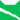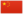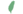# 亮心

Brightheart雷族 武士雷族 猫后雷族 武士雷族 猫后雷族 武士亮崽非官 Brightkit  小亮非官
 亮爪 Brightpaw  亮掌
 夺面 Lostface  無容
 亮心 Brightheart  亮心

Q2844：雷族母猫，自《呼唤野性》登场的角色

## 细节

### 外貌

• 她是一只背部有姜黄色斑块的白色母猫，有着姜黄色的尾巴:62和厚实:7柔软的皮毛。:29她的耳朵碎裂，:108脸上有一道横贯口鼻的巨大伤疤，右脸的毛发被扯掉，:218仅剩一只蓝色的左眼。:7

### 趣闻

• 她尊重云尾不信仰星族的选择。
• 以出版时间来说，她是书中第一只被更改武士名的猫，也是唯一一只由火星改名的猫。:108

## 登场书目

• 呼唤野性 (Q57)[配角][首次登场🐱]
• 寒冰烈火 (Q59)[间接提及]
• 疑云重重 (Q60)[配角]
• 风起云涌 (Q65)[配角]
• 险路惊魂 (Q66)[配角]
• 力挽狂澜 (Q67)[配角]
• 午夜追踪 (Q139)[配角]
• 新月危机 (Q140)[配角]
• 重现家园 (Q141)[配角]
• 星光指路 (Q142)[配角]
• 黄昏战争 (Q143)[配角]
• 日落和平 (Q144)[配角]
• 预视力量 (Q152)[配角]
• 暗河汹涌 (Q153)[配角]
• 驱逐之战 (Q154)[配角]
• 天蚀遮月 (Q155)[配角]
• 暗夜长影 (Q156)[配角]
• 拂晓之光 (Q157)[配角]
• 第四学徒 (Q158)[配角]
• 战声渐近 (Q159)[配角]
• 暗夜密语 (Q160)[配角]
• 月光印记 (Q161)[配角]
• 武士归来 (Q162)[配角]
• 群星之战 (Q163)[配角]
• 学徒探索 (Q170)[配角]
• 雷影交加 (Q171)[配角]
• 天空破碎 (Q172)[配角]
• 极夜无光 (Q173)[配角]
• 烈焰焚河 (Q174)[配角]
• 风暴来袭 (Q175)[猫物闲角]
• 迷失群星 (Q3563)[配角]
• 静默冰融 (Q3579)[配角]
• 叠影重障 (Q3624)[配角]
• 暗由心生 (Q4183)[配角]
• 无星之地 (Q4385)[配角]
• 迷雾明光 (Q4621)[猫物闲角]
• 河翻浪涌 (Q4643)[配角]
• 天穹将倾 (Q4799)[配角]
• 火星的探索 (Q176)[配角]
• 黄牙的秘密 (Q180)[配角]
• 黑莓星的风暴 (Q182)[配角]
• 虎心的阴影 (Q185)[猫物闲角]
• 鸦羽的拷问 (Q186)[配角]
• 松鼠飞的希冀 (Q3561)[对话提及]
• 灰条的誓言 (Q4057)[配角]
• 冬青叶的故事 (Q187)[配角]
• 雾星的征兆 (Q188)[猫物闲角]
• 虎掌的愤怒 (Q190)[猫物闲角]
• 叶池的祈愿 (Q191)[配角]
• 鸽翅的沉默 (Q192)[配角]
• 红尾的恩债 (Q3565)[配角]
• 褐皮的族群 (Q3566)[猫物闲角]
• 蛾翅的秘密 (Q3628)[猫物闲角]
• 黛西的至亲 (Q3629)[猫物闲角]
• 斑毛的反抗 (Q4337)[配角]
• 黑脚的审判 (Q4336)[猫物闲角][仅于回忆]
• 变革之风 (Q4411)[配角]
• 逐出影族 (Q4769)[配角]
• 雷族神偷 (Q5118)
• 武士失踪 (Q207)[配角]
• 族群救星 (Q214)[配角]
• 武士之心 (Q215)[配角]
• 族群的秘密 (Q199)[追溯修订]
• 族群的猫 (Q200)[配角]
• 族群的战争 (Q202)[配角]
• 终极指南 (Q204)[配角]
• 族群决定 (Q4580)[配角]
• 日落之后：正确的选择？ (Q4323)[配角]
• ## 周边

更多资料：亮心/周边

【系列2】炭毛和亮心
• 系列2——炭毛和亮心、黑星和带松鸦羽、乌爪和沙风
• 这对雷族姐妹花历经了艰巨的挑战并取得了胜利。炭毛成为武士的梦想在她弄断腿的那一天破灭了，但她在她巫医的新角色中有着杰出的表现。同时，亮心证明自己是一名伟大武士的决心几乎令她付出了生命的代价——虽然她也将克服她的伤痛，并在族群的荣耀中赢得一席之地。
• 长约7厘米。
• 一组约12.99美金；整套三组约38.97美金。

## 脚注

1. 正体中文版中，错将这句话标为炭毛说的。

## 参考文献

这篇文章基于CC BY-SA 3.0许可使用了猫武士维基（英语）Brightheart一文中的部分内容。